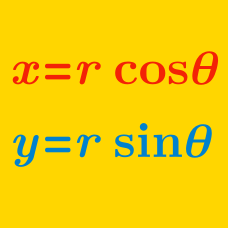Algebra

# Polar Coordinates - Complex Numbers

The complex number $8+8i$ can be expressed in polar form as $a\sqrt{2}(\cos b ^\circ+i\sin b ^\circ),$ where $0 < b < 180$. What is the value of $a+b$?

Let $P$ be the point in the complex plane that corresponds to complex number $z$ and let $O$ be the origin. If $\vert z \vert = 33 \sqrt{2}$ and the angle between the line segment $OP$ and the positive real axis is $\frac{\pi}{4},$ then $z$ can be expressed as $a+bi,$ where $a$ and $b$ are real numbers. What is the value of $a+b?$

Details and assumptions

$i$ is the imaginary number satisfying $i^2 = -1$.

Let $P$ be the point in the complex plane that corresponds to complex number $z,$ and let $O$ be the origin. If $\vert z \vert = 12$ and the angle between the line segment $OP$ and the positive real axis is $\frac{\pi}{b},$ then $z$ can be expressed as $a+12i,$ where $a$ and $b$ are positive integers. What is $a+b?$

Details and assumptions

$i$ is the imaginary number satisfying $i^2 = -1$.

Let $O$ be the origin on the complex plane, and let $P$ be the point representing the complex number $z = 8 + 64i$. If $\theta$ is the angle formed by the line segment $OP$ and the positive part of the real axis of the complex plane, what is the value of $\tan \theta?$

Details and assumptions

$i$ is the imaginary unit, where $i^2=-1$.

Let $P$ be the point in the complex plane that corresponds to complex number $z$ and let $O$ be the origin. If $\vert z \vert = 25 \sqrt{2}$ and the angle between the line segment $OP$ and the positive real axis is $\frac{\pi}{4},$ then $z$ can be expressed as $a+bi,$ where $a$ and $b$ are real numbers. What is the value of $a+b?$

Details and assumptions

$i$ is the imaginary number satisfying $i^2 = -1$.

×